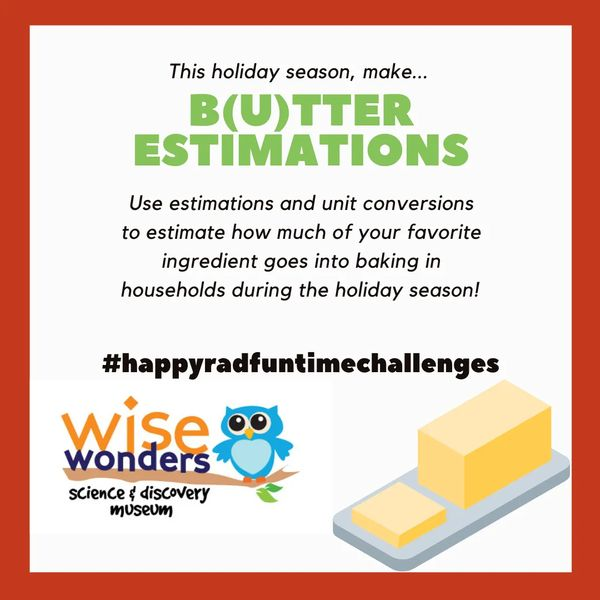# Learn How to Make B(u)tter Estimations!

12/22/2020

### How do "estimations" in math help?

In a lot of math problems, we emphasize getting the correct answer. And a lot of times, getting the correct answer--the exact number, to the exact decimal point, is necessary! But sometimes, an estimation is good enough! It would be very, very hard (almost impossible) to know the exact amount of butter used during the holiday season--but it is definitely possible to know about how much butter is used!

Because each detail (how many households are in the world, how many households use butter during the holidays, how many sticks of butter each of these households use) is an estimation, then the final answer to the problem will be an estimation, as well.

We use estimations to find an answer that gives us the correct order of magnitude. Orders of magnitude give us powers of ten. For instance, when we say that something is "in the millions" it is different from "in the thousands".

For example,

One thousand= 1,000 =10 "to the third power"

and

One million= 1,000,000= 10 "to the sixth power"

So for instance, when we say there are 1 million people who live in Montana, it is really slightly more than this, but the order of magnitude is correct, and gives us a general idea. Orders of magnitude allow us to make comparisons between quantities. This means, even though the exact numbers are estimations, we can know about which factor of ten the quantity probably is.### Check out these links for some more cool math challenges!

"What If" explores hypothetical math and science questions like our butter challenge, and encourages us to go into detail with our calculations:    https://what-if.xkcd.com/

On Matt Parker's Math Puzzles website, a math puzzle is released every other Wednesday:      https://www.think-maths.co.uk/maths-puzzles